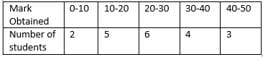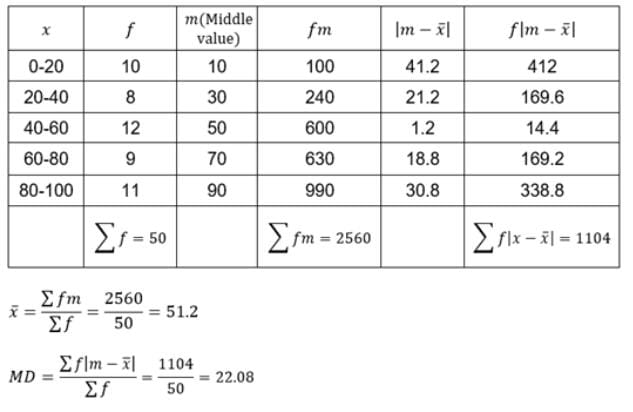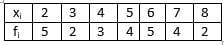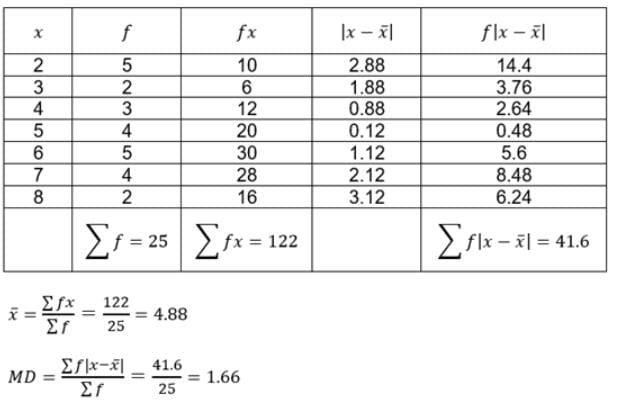# Test: Mean Deviation

## 10 Questions MCQ Test Mathematics For JEE | Test: Mean Deviation

Description
Attempt Test: Mean Deviation | 10 questions in 10 minutes | Mock test for JEE preparation | Free important questions MCQ to study Mathematics For JEE for JEE Exam | Download free PDF with solutions
QUESTION: 1

Solution:
QUESTION: 2

### What is the range of the following data? 23, 45, 34, 21, 89, 45, 47, 91

Solution:

Maximum and minimum value of the data 23, 45, 34, 21, 89, 45, 47, 91 are 21 and 91.
Range = 91 – 21 = 70

QUESTION: 3

### The mean deviation about the mean for the following data:Solution:QUESTION: 4

The mean deviation about the mean for the following data:Solution:QUESTION: 5

The mean deviation about the mean for the following data:
5, 6, 7, 8, 6, 9, 13, 12, 15 is:

Solution:

let, X =5,6,7,8,9,13,12,15.
(5+6+7+8+9+13+12+15)÷9 = 9.
and hence a = 9.
the mean deviation about the mean is summation of |X-a|÷ the total number
i.e , |X-a| = 4,3,2,1,3,0,4,3,6 and the total no. is 9.
hence summation of |X-a| = 26,
the mean deviation is 26 ÷ 9 = 2.89 ans

QUESTION: 6

For ungrouped data, mean deviation about mean is =

Solution:
QUESTION: 7

. ……. about a central value ‘a’ is the mean of the absolute values of the deviations of the observations from ‘a’.

Solution:
QUESTION: 8

The mean deviation of the following data 14, 15, 16, 17, 13 is:

Solution:

Here N= 5 , sigma x = 75
so mean = 15
now taking deviation from mean,( By ignoring signs)
we get sigma deviation from mean = 6
Now applying the formula of mean deviation
M.D.= SIGMA deviation from mean/ n
so M.D.= 6/5
= 1.2

QUESTION: 9

The mean deviation about the mean for the following data 3, 7, 8, 9, 4, 6, 8, 13, 12, 10 is:

Solution:

Arrange data in ascending order,
3,4,6,7,8,8,9,10,12,13
No. of observations = 10
Median = n/2  => 10/2 = 5h observation.
5th observation is 8
Now we calculate mean deviation about median, i.e;
=> ∑∣xi−M∣/10
= {|3-8| +|4-8| +|6-8| +|7-8| +|8-8| +|8-8| +|9-8| +|10-8| +|12-8| +|13-8| }/10
= { 5 + 4 + 2 + 1 + 0 + 0 + 1 + 2 + 4 + 5}/10
= 24/10  => 2.4

QUESTION: 10

The arithmetic mean of the numerical values of the deviations of items from some average value is called the

Solution:

Mean deviation of a data set is the average of the absolute deviations from a central point (Average value).Use Code STAYHOME200 and get INR 200 additional OFF Use Coupon Code